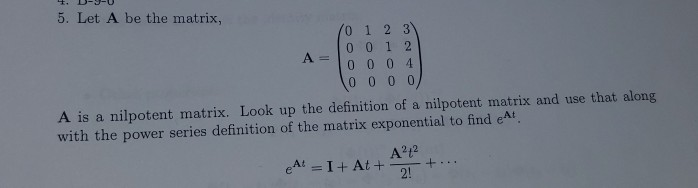# 5. Let A be the matrix, 0 1 2 3 0 0 1 2 A o 0 0 4 A is a nilpotent matrix. Look up the definition...5. Let A be the matrix, 0 1 2 3 0 0 1 2 A o 0 0 4 A is a nilpotent matrix. Look up the definition of a nilpotent matrix and use that along with the power series definition of the matrix exponential to find eAt 2!

The definition of a nilpotent matrix is that , for a square matrix, there exists a positive integer, such thatIn this case, we haveandandIn this case,Hence using the power series definitionUsing thethat we got above, we can write##### Add Answer of: 5. Let A be the matrix, 0 1 2 3 0 0 1 2 A o 0 0 4 A is a nilpotent matrix. Look up the definition...
More Homework Help Questions Additional questions in this topic.